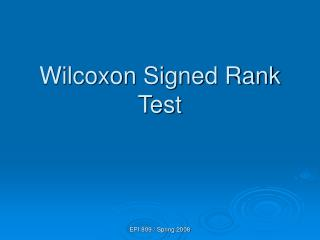Download PresentationWilcoxon Signed Rank Test

# Wilcoxon Signed Rank Test

Download Presentation## Wilcoxon Signed Rank Test

- - - - - - - - - - - - - - - - - - - - - - - - - - - E N D - - - - - - - - - - - - - - - - - - - - - - - - - - -
##### Presentation Transcript

1. Wilcoxon Signed Rank Test EPI 809 / Spring 2008

2. Signed Rank TestExample You work in the finance department. Is the new financial package faster (.05 level)? You collect the following data entry times: UserCurrentNew Donna 9.98 9.88 Santosha 9.88 9.86 Sam 9.90 9.83 Tamika 9.99 9.80 Brian 9.94 9.87 Jorge 9.84 9.84 EPI 809 / Spring 2008 © 1984-1994 T/Maker Co.

3. Wilcoxon Signed Rank Test 1. Tests Probability Distributions of 2 Related Populations 2. Corresponds to t-test for Dependent (Paired) Means 3. Assumptions Random samples; Both populations are continuous; paired samples. Can Use Normal Approximation if #(non-zero differences)  16 EPI 809 / Spring 2008

4. Signed Rank Test Procedure • Obtain Difference Scores, Di= X1i- X2i • Take Absolute Value |Di| and rank them (Do not count Di = 0) • Assign Ranks, Ri, with Smallest = 1 • Calculate range and mean rank for |Di| • Sum ‘+’ Ranks (T+) & ‘-’ Ranks (T-) • Statistic (T+). mean = n(n+1)/4, var = n(n+1)(2n+1)/24 if no ties. EPI 809 / Spring 2008

5. Signed Rank Test Procedure Table method for (n < 16). • Use Table 11 that provides the critical values at significant level. 2. If n ≥ 16, use normal approximation. • Let T0 = n(n+1)/4, If T+ =T0, then T = 0 • Otherwise, if no ties, • If ties, ti =Ri of ties. EPI 809 / Spring 2008

6. Signed Rank Test Procedure (continued) If T > Z1-α/2 then reject H0. Otherwise accept H0. P – value: p = 2 [1 - Φ(T)]. Condition: number of nonzero differences > 15. EPI 809 / Spring 2008

7. Signed Rank Test Example Skin care treatments A vs B • Each subject received two treatments A and B randomly applied to left and right arms. • Measure redness after sunlight exposure of 10 min. • Data: subject 1: X1A, X1B subject 2: X2A, X2B .… EPI 809 / Spring 2008

8. Signed Rank Test Computation Illustration EPI 809 / Spring 2008

9. Testing with positive statistic T+ • T+ = 10*7.5 + 6*19.5 + 2*28 = 248 • E(T+ ) = 40*(40+1)/4 = 410 • Var = 40*(40+1)*(80+1)/24 – [(143-14) + (103-10) + (73-7) +…+ (13-1)]/48 = 5449.75 • T = {|248-10|-.5}/√5449.75 = 2.19 • P = 2*[1- Φ(2.19)] = 0.029. EPI 809 / Spring 2008

10. Signed Rank TestExample You work in the finance department. Is the new financial package faster (.05 level)? You collect the following data entry times: UserCurrentNew Donna 9.98 9.88 Santosha 9.88 9.86 Sam 9.90 9.83 Tamika 9.99 9.80 Brian 9.94 9.87 Jorge 9.84 9.84 EPI 809 / Spring 2008 © 1984-1994 T/Maker Co.

11. Signed Rank Test Computation Table EPI 809 / Spring 2008

12. Signed Rank Test Solution • H0: Identical Distrib. • Ha: Current Shifted Right •  = .05 (one-sided) • n’ = 5 (not 6; 1 elim.) • Critical Value(s): Test Statistic: Decision: Conclusion: Do Not Reject Reject TL Tu EPI 809 / Spring 2008

13. Wilcoxon Signed Rank Table (Portion) EPI 809 / Spring 2008

14. Signed Rank Test Solution • H0: Identical Distrib. • Ha: Current Shifted Right •  = .05 (one-sided) • n’ = 5 (not 6; 1 elim.) • Critical Value(s): Test Statistic: Decision: Conclusion: Do Not Reject 0 15 EPI 809 / Spring 2008

15. Signed Rank Test Solution • H0: Identical Distrib. • Ha: Current Shifted Right •  = .05 (one-sided) • n’ = 5 (not 6; 1 elim.) • Critical Value(s): Test Statistic: Decision: Conclusion: Since one-sided Test & Current Shifted Right, Use T+ Do Not Reject 0 15 EPI 809 / Spring 2008

16. Signed Rank Test Solution • H0: Identical Distrib. • Ha: Current Shifted Right •  = .05 (one-sided) • n’ = 5 (not 6; 1 elim.) • Critical Value(s): Test Statistic: Conclusion: Since One-sided Test & Current Shifted Right, Use T+T+ = 15 Do Not Reject Border line at  = .05 0 15 EPI 809 / Spring 2008

17. Kruskal-Wallis test • A k-sample non-parametric test on the means (k > 2). • Pool observations together N = ∑ni andassign ranks to individuals. • Compute the rank sum Ri for each sample. • Test statistic Chi-squares with df = (k-1) one tail prob. Compare with χ2k-1, 1-α • Should only be used if smallest ni ≥ 5. EPI 809 / Spring 2008

18. Kruskal-Wallis test statistic • If no ties. • If ties. Denote ti, g as before. EPI 809 / Spring 2008

19. Conclusion • 1. Distinguished Parametric & Nonparametric Test Procedures • 2. Explained a Variety of Nonparametric Test Procedures • 3. Solved Hypothesis Testing Problems Using Nonparametric Tests EPI 809 / Spring 2008

20. Summary EPI 809 / Spring 2008